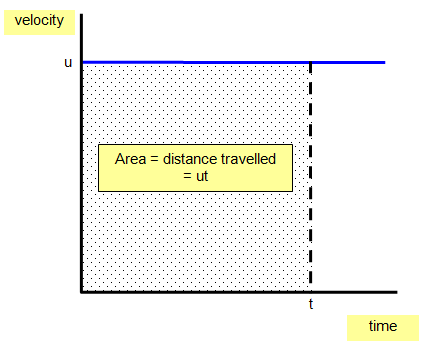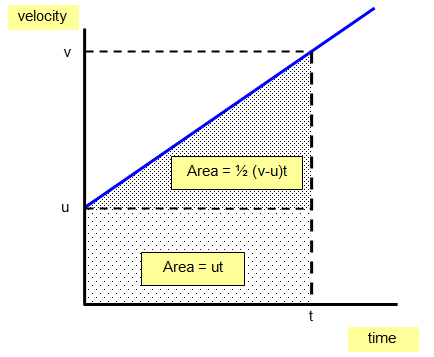Equations of motion

In studying the motion of objects it is often helpful to use an equation to work out the velocity, acceleration or the distance travelled.

We use the following letters to represent certain quantities:

Distance travelled s measured in metres (m)
Time taken t measured in seconds (s)
The velocity at the start (called initial velocity) u measured in m/s
The velocity at the end (called the final velocity) v measured in m/s
The acceleration of the object a measured in m/s2

1. Non accelerated motion – that is motion at a constant velocityThe area under the line of the velocity –time graph is the distance travelled by the object in the time t.

For example u = 20m/s and t = 300 s
Distance (s) = ut = 20 x 300 = 6000 m

The equation for non accelerated motion is:

Distance (s) = velocity (u or v) x time (t)      s = vt

2. Accelerated motion

Acceleration (a) = [change in velocity]/time = [v-u]/t
or
a = [v-u]/t

Another version is:      v = u + atDistance travelled = area under the line = ut + ˝ (v-u)t

But acceleration = (v-u)/t and so (v-u) = at therefore:

Distance travelled (s) = ut + ˝ (v-u)t = ut + ˝ [at]t = ut + ˝ at2

s = ut + ˝at2

If the object starts from rest u = 0, and so the equation becomes:

s = ˝at2

Another useful equation is:

v2 = u2 + 2as

USING EQUATIONS

This section is designed to help you work out some of the problems using the equations of motion.

CONSTANT VELOCITY
1. s = vt

CONSTANT ACCELERATION
2. v = u + at
3. s = ut + ˝at2
4. average velocity = [v + u]/2
5. v2 = u2 + 2as

If you need to use any of these equations to work out problems the way to do it is this:

(a) write down what you are given, usually three things
(b) look for the equation that contains these three things and the quantity that you are trying to find
(c) put the numbers in the CORRECT equation and work it out

You will need to know how to rearrange equations to make different quantities the subject of the equation.

You might like to try using the triangle rule if there are only three quantities in the equation but some of them are more complex than this.

Example problems
1. A horse accelerates steadily from rest at 4 m/s2 for 3s.
What is its final velocity and how far has it travelled?
(a) Initial velocity (u) = 0 Final velocity (v) = ?
Acceleration (a) = 4 m/s2 Time (t) = 3 s.
Known quantities u,a and t. Unknown v.
Correct equation is:   v = u + at
Final velocity (v) = 0 + 4x3 = 12 m/s
(b) Correct equation is s = ut + ˝at2
Distance (s) = 0x3 + 0.5x4x9 = 18 m

2. A car travelling at 30 m/s accelerates steadily at 5 m/s2 for a distance of 70 m. What is the final velocity of the car?
Known quantities u,a and s. Unknown v.
Correct equation is v2 = u2 + 2as
Final velocity2 (v2) = 30x30 + 2x5x70
Therefore v = 40 m/s

© Keith Gibbs 2016